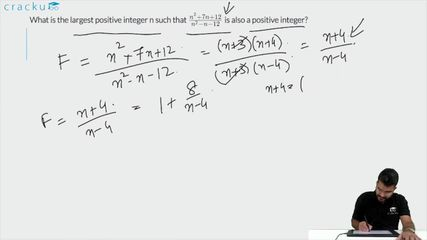Question 69

# What is the largest positive integer n such that $$\frac{n^2 + 7n + 12}{n^2 - n - 12}$$ is also a positive integer?

Solution

$$\ \frac{\ n^2+3n+4n+12}{n^2-4n+3n-12}$$

=$$\ \frac{\ n^{ }\left(n+3\right)+4\left(n+3\right)}{n^{ }\left(n-4\right)+3\left(n-4\right)}$$

=$$\ \frac{\left(\ n+4\right)\left(n+3\right)}{\left(n-4\right)\left(n+3\right)}$$

=$$\ \frac{\left(\ n+4\right)}{\left(n-4\right)}$$

=$$\ \frac{\left(\ n-4\right)+8}{\left(n-4\right)}$$

=$$\ 1+\frac{8}{\left(n-4\right)}$$ which will be maximum when n-4 =8

n=12

### View Video Solution• All Quant CAT Formulas and shortcuts PDF
• 30+ CAT previous papers with solutions PDF

##### GOPAL IDHOLE

4 months, 3 weeks ago

where does that 8 come from??

##### Shamita Sharma

3 years, 5 months ago

I could not understand this